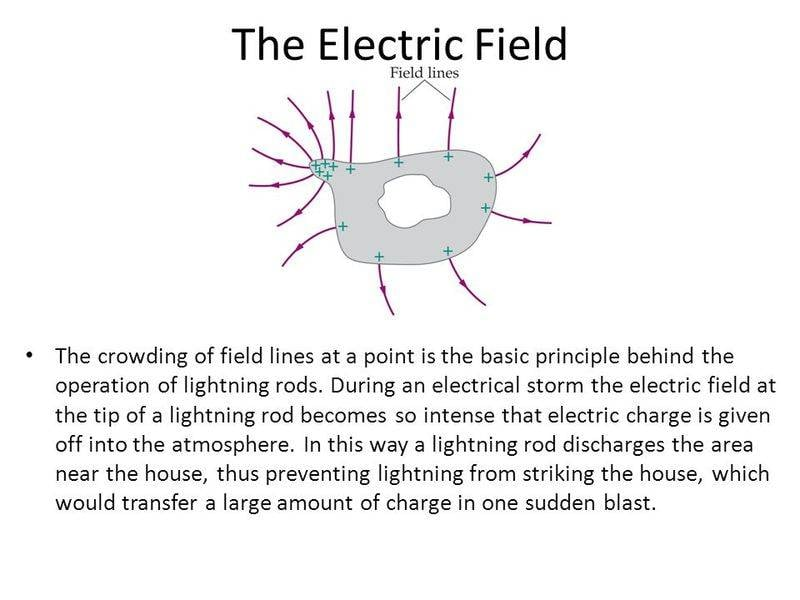# Breakdown Voltage Depends on Field Uniformity?

Which is higher, the breakdown voltage if the electric field is uniform or the breakdown voltage if the electric field is non-uniform ?

kuruman
Homework Helper
Gold Member
The question is a bit confusing. As I understand it, the breakdown voltage is a specific threshold value not related to what the field looks like. Of course a "real" field may be pretty close to uniform in a certain region of space, but may very well be non-uniform in some other region of space. As you increase the field everywhere, breakdown will occur where the field happens to be the strongest because that's where the threshold value will be reached first.

The simplest models of breakdown do assume uniform field but that's not necessarily what any reality actually is. Models are approximations for us stupid humans, not because reality must follow the model exactly.

This is where/why you can apply these ideals to a FEA simulation - within each mesh element, the assumption is exactly that kind of uniformity.

You can get some complicated results in reality and in an FEA simulation because there may be some parts (if physical aspects are well modeled) that will cause a small piece to breakdown and then cascade to more complicated breakdown phenomena like the fractal curves of a Litchtenberg figure. The problem is usually you don't know, and have no way to find out, the specific, small variations in the material that create weak spots for that lead to such things. There's also random processes involve that assure it might not happen the same way twice.

This requires FEA that can model that kind of variability per mesh element in the "proper way" (e.g. defects per cm^3 or similar). It gets complicated for physical phenomena where the act of something happening (e.g. conduction from point A to point B) itself changes the probability of some other subsequent things happening (e.g. conduction from point B to point C, B to D, B to E, B to F... B to ZZZZZ). This s the nature of fractal and chaotic behaviors - deterministic models and behaviors becomes the wrong question. Breakdown phenomena have this kind of effect which is why there is no simple algebraic formula for the relationship of breakdown current vs. applied voltage. Instead you have to rely on empirical data like Paschen Law which are still only approximate.

https://en.wikipedia.org/wiki/Lichtenberg_figure

https://en.wikipedia.org/wiki/Lightning_strike

https://en.wikipedia.org/wiki/Paschen's_law

•anorlunda
Svein•